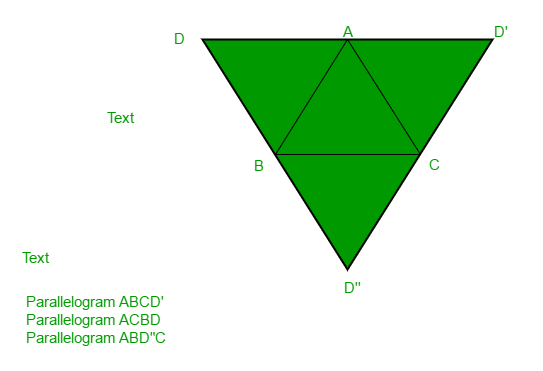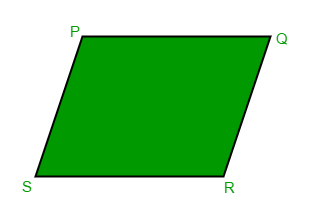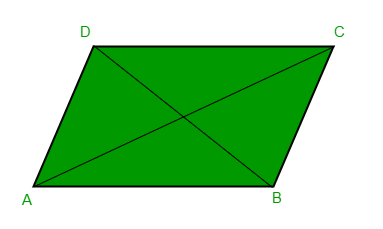GFG App
Open AppBrowser
Continue

# Find the Missing Point of Parallelogram

Given three coordinate points A, B and C, find the missing point D such that ABCD can be a parallelogram.
Examples :

```Input : A = (1, 0)
B = (1, 1)
C = (0, 1)
Output : 0, 0
Explanation:
The three input points form a unit
square with the point (0, 0)

Input : A = (5, 0)
B = (1, 1)
C = (2, 5)
Output : 6, 4```

As shown in below diagram, there can be multiple possible outputs, we need to print any one of them.A quadrilateral is said to be a parallelogram if its opposite sides are parallel and equal in length.As we’re given three points of the parallelogram, we can find the slope of the missing sides as well as their lengths.
The algorithm can be explained as follows
Let R be the missing point. Now from definition, we have

• Length of PR = Length of QS = L1 (Opposite sides are equal)
• Slope of PR = Slope of QS = M1 (Opposite sides are parallel)
• Length of PQ = Length of RS = L2 (Opposite sides are equal)
• Slope of PQ= Slope of RS = M2 (Opposite sides are parallel)

Thus we can find the points at a distance L1 from P having slope M1 as mentioned in below article :
Find points at a given distance on a line of given slope.
Now one of the points will satisfy the above conditions which can easily be checked (using either condition 3 or 4)

Below is the implementation of the above approach:

## C++

 `// C++ program to find missing point of a` `// parallelogram` `#include ` `using` `namespace` `std;`   `// struct to represent a co-ordinate point` `struct` `Point {` `    ``float` `x, y;` `    ``Point()` `    ``{` `        ``x = y = 0;` `    ``}` `    ``Point(``float` `a, ``float` `b)` `    ``{` `        ``x = a, y = b;` `    ``}` `};`   `// given a source point, slope(m) of line` `// passing through it this function calculates` `// and return two points at a distance l away` `// from the source` `pair findPoints(Point source,` `                              ``float` `m, ``float` `l)` `{` `    ``Point a, b;`   `    ``// slope is 0` `    ``if` `(m == 0) {` `        ``a.x = source.x + l;` `        ``a.y = source.y;`   `        ``b.x = source.x - l;` `        ``b.y = source.y;` `    ``}`   `    ``// slope if infinity` `    ``else` `if` `(m == std::numeric_limits<``float``>::max()) {` `        ``a.x = source.x;` `        ``a.y = source.y + l;`   `        ``b.x = source.x;` `        ``b.y = source.y - l;` `    ``}`   `    ``// normal case` `    ``else` `{` `        ``float` `dx = (l / ``sqrt``(1 + (m * m)));` `        ``float` `dy = m * dx;` `        ``a.x = source.x + dx, a.y = source.y + dy;` `        ``b.x = source.x - dx, b.y = source.y - dy;` `    ``}`   `    ``return` `pair(a, b);` `}`   `// given two points, this function calculates` `// the slope of the line/ passing through the` `// points` `float` `findSlope(Point p, Point q)` `{` `    ``if` `(p.y == q.y)` `        ``return` `0;` `    ``if` `(p.x == q.x)` `        ``return` `std::numeric_limits<``float``>::max();` `    ``return` `(q.y - p.y) / (q.x - p.x);` `}`   `// calculates the distance between two points` `float` `findDistance(Point p, Point q)` `{` `    ``return` `sqrt``(``pow``((q.x - p.x), 2) + ``pow``((q.y - p.y), 2));` `}`   `// given three points, it prints a point such` `// that a parallelogram is formed` `void` `findMissingPoint(Point a, Point b, Point c)` `{` `    ``// calculate points originating from a` `    ``pair d = findPoints(a, findSlope(b, c),` `                                      ``findDistance(b, c));`   `    ``// now check which of the two points satisfy` `    ``// the conditions` `    ``if` `(findDistance(d.first, c) == findDistance(a, b))` `        ``cout << d.first.x << ``", "` `<< d.first.y << endl;` `    ``else` `        ``cout << d.second.x << ``", "` `<< d.second.y << endl;` `}`   `// Driver code` `int` `main()` `{` `    ``findMissingPoint(Point(1, 0), Point(1, 1), Point(0, 1));` `    ``findMissingPoint(Point(5, 0), Point(1, 1), Point(2, 5));` `    ``return` `0;` `}`

## Java

 `// Java program to find missing point of a` `// parallelogram` `import` `java.util.*;`   `// struct to represent a co-ordinate point` `class` `Point {` `  ``public` `double` `x, y;` `  ``public` `Point()` `  ``{` `    ``x = ``0``;` `    ``y = ``0``;` `  ``}`   `  ``public` `Point(``double` `a, ``double` `b)` `  ``{` `    ``x = a;` `    ``y = b;` `  ``}` `};`   `class` `GFG {` `  ``// given a source point, slope(m) of line` `  ``// passing through it this function calculates` `  ``// and return two points at a distance l away` `  ``// from the source` `  ``static` `Point[] findPoints(Point source, ``double` `m,` `                            ``double` `l)` `  ``{` `    ``Point a = ``new` `Point();` `    ``Point b = ``new` `Point();`   `    ``// slope is 0` `    ``if` `(m == ``0``) {` `      ``a.x = source.x + l;` `      ``a.y = source.y;`   `      ``b.x = source.x - l;` `      ``b.y = source.y;` `    ``}`   `    ``// slope if infinity` `    ``else` `if` `(m == Double.MAX_VALUE) {` `      ``a.x = source.x;` `      ``a.y = source.y + l;`   `      ``b.x = source.x;` `      ``b.y = source.y - l;` `    ``}`   `    ``// normal case` `    ``else` `{` `      ``double` `dx = (l / Math.sqrt(``1` `+ (m * m)));` `      ``double` `dy = m * dx;` `      ``a.x = source.x + dx;` `      ``a.y = source.y + dy;` `      ``b.x = source.x - dx;` `      ``b.y = source.y - dy;` `    ``}`   `    ``Point[] res = { a, b };` `    ``return` `res;` `  ``}`   `  ``// given two points, this function calculates` `  ``// the slope of the line/ passing through the` `  ``// points` `  ``static` `double` `findSlope(Point p, Point q)` `  ``{` `    ``if` `(p.y == q.y)` `      ``return` `0``;` `    ``if` `(p.x == q.x)` `      ``return` `Double.MAX_VALUE;` `    ``return` `(q.y - p.y) / (q.x - p.x);` `  ``}`   `  ``// calculates the distance between two points` `  ``static` `double` `findDistance(Point p, Point q)` `  ``{` `    ``return` `Math.sqrt(Math.pow((q.x - p.x), ``2``)` `                     ``+ Math.pow((q.y - p.y), ``2``));` `  ``}`   `  ``// given three points, it prints a point such` `  ``// that a parallelogram is formed` `  ``static` `void` `findMissingPoint(Point a, Point b, Point c)` `  ``{` `    ``// calculate points originating from a` `    ``Point[] d = findPoints(a, findSlope(b, c),` `                           ``findDistance(b, c));`   `    ``// now check which of the two points satisfy` `    ``// the conditions` `    ``if` `(findDistance(d[``0``], c) == findDistance(a, b))` `      ``System.out.println(d[``0``].x + ``", "` `+ d[``0``].y);` `    ``else` `      ``System.out.println(d[``1``].x + ``", "` `+ d[``1``].y);` `  ``}`   `  ``// Driver code` `  ``public` `static` `void` `main(String[] args)` `  ``{` `    ``findMissingPoint(``new` `Point(``1``, ``0``), ``new` `Point(``1``, ``1``),` `                     ``new` `Point(``0``, ``1``));` `    ``findMissingPoint(``new` `Point(``5``, ``0``), ``new` `Point(``1``, ``1``),` `                     ``new` `Point(``2``, ``5``));` `  ``}` `}`   `// This code is contributed by phasing17`

## Python3

 `# Python program to find missing point of a` `# parallelogram`   `import` `math as Math` `FLOAT_MAX ``=` `3.40282e``+``38`   `# given a source point, slope(m) of line` `# passing through it this function calculates` `# and return two points at a distance l away` `# from the source`     `def` `findPoints(source, m, l):` `    ``a ``=` `[``0``] ``*` `(``2``)` `    ``b ``=` `[``0``] ``*` `(``2``)`   `    ``# slope is 0` `    ``if` `(m ``=``=` `0``):` `        ``a[``0``] ``=` `source[``0``] ``+` `l` `        ``a[``1``] ``=` `source[``1``]`   `        ``b[``0``] ``=` `source[``0``] ``-` `l` `        ``b[``1``] ``=` `source[``1``]` `    ``# slope if infinity` `    ``elif` `(m ``=``=` `FLOAT_MAX):` `        ``a[``0``] ``=` `source[``0``]` `        ``a[``1``] ``=` `source[``1``] ``+` `l`   `        ``b[``0``] ``=` `source[``0``]` `        ``b[``1``] ``=` `source[``1``] ``-` `l` `    ``# normal case` `    ``else``:` `        ``dx ``=` `(l ``/` `((``1` `+` `(m ``*` `m)) ``*``*` `0.5``))` `        ``dy ``=` `m ``*` `dx` `        ``a[``0``] ``=` `source[``0``] ``+` `dx` `        ``a[``1``] ``=` `source[``1``] ``+` `dy` `        ``b[``0``] ``=` `source[``0``] ``-` `dx` `        ``b[``1``] ``=` `source[``1``] ``-` `dy`   `    ``return` `[a, b]`     `# given two points, this function calculates` `# the slope of the line/ passing through the` `# points` `def` `findSlope(p, q):` `    ``if` `(p[``1``] ``=``=` `q[``1``]):` `        ``return` `0` `    ``if` `(p[``0``] ``=``=` `q[``0``]):` `        ``return` `FLOAT_MAX` `    ``return` `(q[``1``] ``-` `p[``1``]) ``/` `(q[``0``] ``-` `p[``0``])`     `# calculates the distance between two points` `def` `findDistance(p, q):` `    ``return` `Math.sqrt(Math.``pow``((q[``0``] ``-` `p[``0``]), ``2``) ``+` `Math.``pow``((q[``1``] ``-` `p[``1``]), ``2``))`     `# given three points, it prints a point such` `# that a parallelogram is formed` `def` `findMissingPoint(a, b, c):` `    ``# calculate points originating from a` `    ``d ``=` `findPoints(a, findSlope(b, c), findDistance(b, c))`   `    ``# now check which of the two points satisfy` `    ``# the conditions` `    ``if` `(findDistance(d[``0``], c) ``=``=` `findDistance(a, b)):` `        ``print``(f``"{(int)(d)}, {(int)(d)}"``)`   `    ``else``:` `        ``print``(f``"{(int)(d)}, {(int)(d)}"``)`     `# Driver code` `Point1 ``=` `[``1``, ``0``]` `Point2 ``=` `[``1``, ``1``]` `Point3 ``=` `[``0``, ``1``]` `findMissingPoint(Point1, Point2, Point3)`   `Point1 ``=` `[``5``, ``0``]` `Point2 ``=` `[``1``, ``1``]` `Point3 ``=` `[``2``, ``5``]` `findMissingPoint(Point1, Point2, Point3)`   `# The code is contributed by Saurabh Jaiswal`

## C#

 `// C# program to find missing point of a` `// parallelogram` `using` `System;` `using` `System.Collections.Generic;`   `// struct to represent a co-ordinate point` `class` `Point {` `  ``public` `double` `x, y;` `  ``public` `Point()` `  ``{` `    ``x = 0;` `    ``y = 0;` `  ``}`   `  ``public` `Point(``double` `a, ``double` `b)` `  ``{` `    ``x = a;` `    ``y = b;` `  ``}` `};`   `class` `GFG` `{`   `  ``// given a source point, slope(m) of line` `  ``// passing through it this function calculates` `  ``// and return two points at a distance l away` `  ``// from the source` `  ``static` `Point[] findPoints(Point source, ``double` `m,` `                            ``double` `l)` `  ``{` `    ``Point a = ``new` `Point();` `    ``Point b = ``new` `Point();`   `    ``// slope is 0` `    ``if` `(m == 0) {` `      ``a.x = source.x + l;` `      ``a.y = source.y;`   `      ``b.x = source.x - l;` `      ``b.y = source.y;` `    ``}`   `    ``// slope if infinity` `    ``else` `if` `(m == Double.MaxValue) {` `      ``a.x = source.x;` `      ``a.y = source.y + l;`   `      ``b.x = source.x;` `      ``b.y = source.y - l;` `    ``}`   `    ``// normal case` `    ``else` `{` `      ``double` `dx = (l / Math.Sqrt(1 + (m * m)));` `      ``double` `dy = m * dx;` `      ``a.x = source.x + dx;` `      ``a.y = source.y + dy;` `      ``b.x = source.x - dx;` `      ``b.y = source.y - dy;` `    ``}`   `    ``Point[] res = { a, b };` `    ``return` `res;` `  ``}`   `  ``// given two points, this function calculates` `  ``// the slope of the line/ passing through the` `  ``// points` `  ``static` `double` `findSlope(Point p, Point q)` `  ``{` `    ``if` `(p.y == q.y)` `      ``return` `0;` `    ``if` `(p.x == q.x)` `      ``return` `Double.MaxValue;` `    ``return` `(q.y - p.y) / (q.x - p.x);` `  ``}`   `  ``// calculates the distance between two points` `  ``static` `double` `findDistance(Point p, Point q)` `  ``{` `    ``return` `Math.Sqrt(Math.Pow((q.x - p.x), 2)` `                     ``+ Math.Pow((q.y - p.y), 2));` `  ``}`   `  ``// given three points, it prints a point such` `  ``// that a parallelogram is formed` `  ``static` `void` `findMissingPoint(Point a, Point b, Point c)` `  ``{` `    ``// calculate points originating from a` `    ``Point[] d = findPoints(a, findSlope(b, c),` `                           ``findDistance(b, c));`   `    ``// now check which of the two points satisfy` `    ``// the conditions` `    ``if` `(findDistance(d, c) == findDistance(a, b))` `      ``Console.WriteLine(d.x + ``", "` `+ d.y);` `    ``else` `      ``Console.WriteLine(d.x + ``", "` `+ d.y);` `  ``}`   `  ``// Driver code` `  ``public` `static` `void` `Main(``string``[] args)` `  ``{` `    ``findMissingPoint(``new` `Point(1, 0), ``new` `Point(1, 1),` `                     ``new` `Point(0, 1));` `    ``findMissingPoint(``new` `Point(5, 0), ``new` `Point(1, 1),` `                     ``new` `Point(2, 5));` `  ``}` `}`   `// This code is contributed by phasing17`

## Javascript

 `// JavaScript program to find missing point of a` `// parallelogram`   `const FLOAT_MAX = 3.40282e+38;`   `// given a source point, slope(m) of line` `// passing through it this function calculates` `// and return two points at a distance l away` `// from the source` `function` `findPoints(source, m, l)` `{` `    ``let a = ``new` `Array(2);` `    ``let b = ``new` `Array(2);`   `    ``// slope is 0` `    ``if` `(m == 0) {` `        ``a = source + l;` `        ``a = source;`   `        ``b = source - l;` `        ``b = source;` `    ``}` `    ``// slope if infinity` `    ``else` `if` `(m == FLOAT_MAX) {` `        ``a = source;` `        ``a = source + l;`   `        ``b = source;` `        ``b = source - l;` `    ``}` `    ``// normal case` `    ``else` `{` `        ``let dx = (l / Math.sqrt(1 + (m * m)));` `        ``let dy = m * dx;` `        ``a = source + dx, a = source + dy;` `        ``b = source - dx, b = source - dy;` `    ``}`   `    ``return` `[a, b];` `}`   `// given two points, this function calculates` `// the slope of the line/ passing through the` `// points` `function` `findSlope(p, q)` `{` `    ``if` `(p == q){` `        ``return` `0;` `    ``}` `    ``if` `(p == q){` `        ``return` `FLOAT_MAX;` `    ``}` `    ``return` `(q - p) / (q - p);` `}`   `// calculates the distance between two points` `function` `findDistance(p, q)` `{` `    ``return` `Math.sqrt(Math.pow((q - p), 2) + Math.pow((q - p), 2));` `}`   `// given three points, it prints a point such` `// that a parallelogram is formed` `function` `findMissingPoint(a, b, c)` `{` `    ``// calculate points originating from a` `    ``let d = findPoints(a, findSlope(b, c), findDistance(b, c));`   `    ``// now check which of the two points satisfy` `    ``// the conditions` `    ``if` `(findDistance(d, c) === findDistance(a, b)){` `        ``console.log(d, ``","``, d);` `    ``}` `    ``else``{` `        ``console.log(d, ``","``, d);` `    ``}     ` `}`   `// Driver code` `{` `    ``let Point1 = [1, 0];` `    ``let Point2 = [1, 1];` `    ``let Point3 = [0, 1];` `    ``findMissingPoint(Point1, Point2, Point3);` `    `  `    ``Point1 = [5, 0];` `    ``Point2 = [1, 1];` `    ``Point3 = [2, 5];` `    ``findMissingPoint(Point1, Point2, Point3);` `}`   `// The code is contributed by Gautam goel (gautamgoel962)`

Output :

```0, 0
6, 4```

Time Complexity: O(log(log n)) since using inbuilt sqrt and log functions
Auxiliary Space: O(1)

Alternative Approach:Since the opposite sides are equal, AD = BC and AB = CD, we can calculate the co-ordinates of the missing point (D) as:

```AD = BC
(Dx - Ax, Dy - Ay) = (Cx - Bx, Cy - By)
Dx = Ax + Cx - Bx
Dy = Ay + Cy - By```

References: https://math.stackexchange.com/questions/887095/find-the-4th-vertex-of-the-parallelogram
Below is the implementation of above approach:

## C++

 `// C++ program to find missing point` `// of a parallelogram` `#include ` `using` `namespace` `std;`   `// main method` `int` `main()` `{` `   ``int` `ax = 5, ay = 0; ``//coordinates of A` `   ``int` `bx = 1, by = 1; ``//coordinates of B` `   ``int` `cx = 2, cy = 5; ``//coordinates of C` `    ``cout << ax + cx - bx << ``", "` `         ``<< ay + cy - by;` `    ``return` `0;` `}`

## Java

 `// Java program to ` `// find missing point` `// of a parallelogram` `import` `java.io.*;`   `class` `GFG ` `{` `public` `static` `void` `main (String[] args) ` `{`   `    ``int` `ax = ``5``, ay = ``0``; ``//coordinates of A` `    ``int` `bx = ``1``, by = ``1``; ``//coordinates of B` `    ``int` `cx = ``2``, cy = ``5``; ``//coordinates of C` `    ``System.out.println(ax + (cx - bx) + ``", "` `+` `                       ``ay + (cy - by));` `}` `}`   `// This code is contributed by m_kit`

## Python 3

 `# Python 3 program to find missing point` `# of a parallelogram`   `# Main method` `if` `__name__ ``=``=` `"__main__"``:` `    `  `    ``# coordinates of A` `    `  `    ``ax, ay ``=` `5``, ``0` `    `  `    ``# coordinates of B` `    `  `    ``bx ,by ``=` `1``, ``1` `    `  `    ``# coordinates of C` `    `  `    ``cx ,cy ``=` `2``, ``5` `    `  `    ``print``(ax ``+` `cx ``-` `bx , ``","``, ay ``+` `cy ``-` `by)`   `# This code is contributed by Smitha`

## C#

 `// C# program to ` `// find missing point` `// of a parallelogram` `using` `System;`   `class` `GFG` `{` `    ``static` `public` `void` `Main ()` `    ``{` `        ``int` `ax = 5, ay = 0; ``//coordinates of A` `        ``int` `bx = 1, ``by` `= 1; ``//coordinates of B` `        ``int` `cx = 2, cy = 5; ``//coordinates of C` `        ``Console.WriteLine(ax + (cx - bx) + ``", "` `+` `                             ``ay + (cy - ``by``));` `    ``}` `}`   `// This code is contributed by ajit`

## PHP

 ``

## Javascript

 ``

Output:

`6, 4`

Time Complexity: O(1)
Auxiliary Space: O(1)

This article is contributed by Aarti_Rathi and Ashutosh Kumar 😀 and Abhishek Sharma. If you like GeeksforGeeks and would like to contribute, you can also write an article using write.geeksforgeeks.org or mail your article to review-team@geeksforgeeks.org. See your article appearing on the GeeksforGeeks main page and help other Geeks.